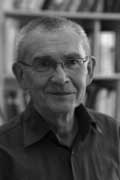Amidst all LHC-noise, Yuri I. Manin arXived today an interesting paper Cyclotomy and analytic geometry over $\mathbb{F}_1$.

The paper gives a nice survey of the existent literature and focusses on the crucial role of roots of unity in the algebraic geometry over the non-existent field with one element $\mathbb{F}_1$ (in French called ‘F-un’). I have tried to do a couple of posts on F-un some time ago but now realize, reading Manin’s paper, I may have given up way too soon…

At several places in the paper, Manin hints at a possible noncommutative geometry over $\mathbb{F}_1$ :

This is the appropriate place to stress that in a wider context of Toen-Vaqui ‘Au-dessous de Spec Z’, or eventually in noncommutative $\mathbb{F}_1$-geometry, teh spectrum of $\mathbb{F}_1$ loses its privileged position as a final object of a geometric category. For example, in noncommutative geometry, or in an appropriate category of stacks, the quotient of this spectrum modulo the trivial action of a group must lie below this spectrum.

Soule’s algebras $\mathcal{A}_X$ are a very important element of the structure, in particular, because they form a bridge to Arakelov geometry. Soule uses concrete choices of them in order to produce ‘just right’ supply of morphisms, without a priori constraining these choices formally. In this work, we use these algebras and their version also to pave a way to the analytic (and possibly non-commutative) geometry over $\mathbb{F}_1$.

Back when I was writing the first batch of F-un posts, I briefly contemplated the possibility of a noncommutative geometry over $\mathbb{F}_1$, but quickly forgot about it because I thought it would be forced to reduce to commutative geometry.

Here is the quick argument : noncommutative geometry is really the study of coalgebras (see for example my paper or if you prefer more trustworthy sources the Kontsevich-Soibelman paper). Now, unless I made a mistake, I think all coalgebras over $\mathbb{F}_1$ must be co-commutative (even group-like), so reducing to commutative geometry.

Surely, I’m missing something…

Published in absolute geometry stories